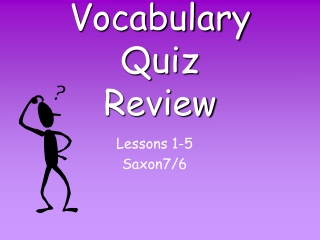DownloadDownload PresentationVocabulary Quiz Review

# Vocabulary Quiz Review

Download Presentation## Vocabulary Quiz Review

- - - - - - - - - - - - - - - - - - - - - - - - - - - E N D - - - - - - - - - - - - - - - - - - - - - - - - - - -
##### Presentation Transcript

1. Vocabulary Quiz Review Lessons 1-5 Saxon7/6

2. Addends Two or more numbers that are added to find a sum

3. Commutative Property of Addition Changing the order of addends does not change their sum. Ex. A + B = B + A

4. Difference The result of subtraction

5. Fact Family A group of three numbers related by addition and subtraction or by multiplication and division Ex. 2+3=5 3+2=5 5-2=3 5-3=2

6. Identity Property of Addition The sum of any number and 0 is equal to the initial number. Ex. 7 + 0 = 7

7. Inverse Operations Operations that “undo” one another Ex. Addition/Subtraction Multiplication/Division

8. Minuend A number from which another number is subtracted Ex. A - 3 = 2

9. Subtrahend A number that is subtracted Ex. 7 - A = 2

10. Sum The result of addition

11. Commutative Property of Multiplication Changing the order of factors does not change their product. Ex. A x B = B x A

12. Dividend A number that is divided

13. Divisor A number by which another number is divided

14. Factors Two or more numbers that are multiplied

15. Identity Property of Multiplication The product of any number and 1 is equal to the initial number. Ex. 7 x 1 = 7

16. Product The result of multiplication

17. Quotient The result of division

18. Remainder An amount left after division

19. Zero Property of Multiplication Zero times any number is zero Ex. 0 x 4 = 0

20. Associative Property of Addition The grouping of addends does not affect their sum. Ex. (a + b) + c = a + (b + c)

21. Associative Property of Multiplication The grouping of factors does not affect their product. Ex. (a x b) x c = a x (b x c)

22. Study!!!!!!

23. This powerpoint was kindly donated to www.worldofteaching.com http://www.worldofteaching.com is home to over a thousand powerpoints submitted by teachers. This is a completely free site and requires no registration. Please visit and I hope it will help in your teaching.